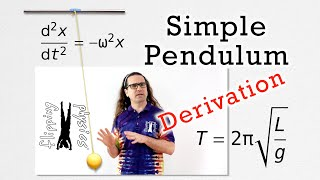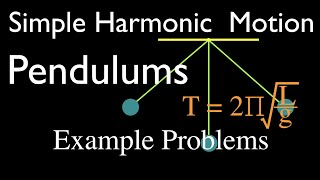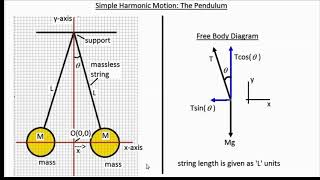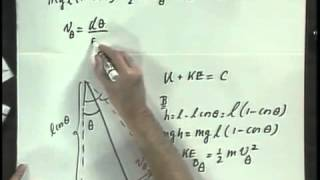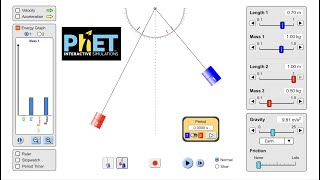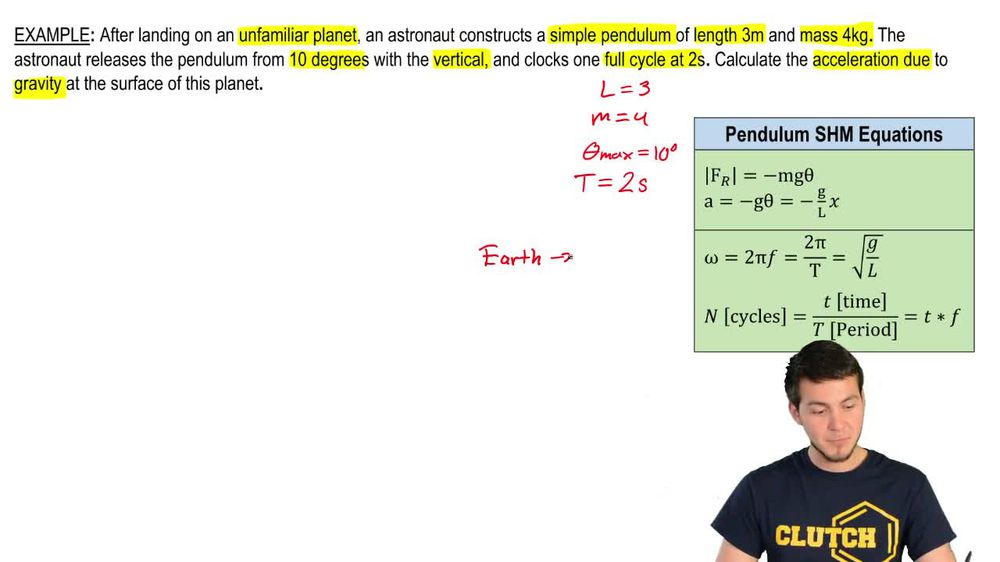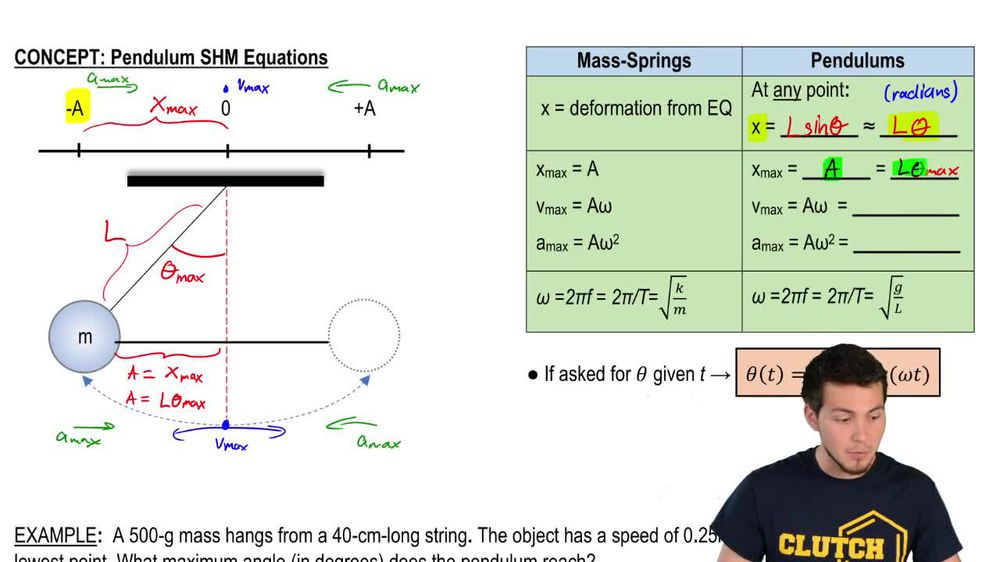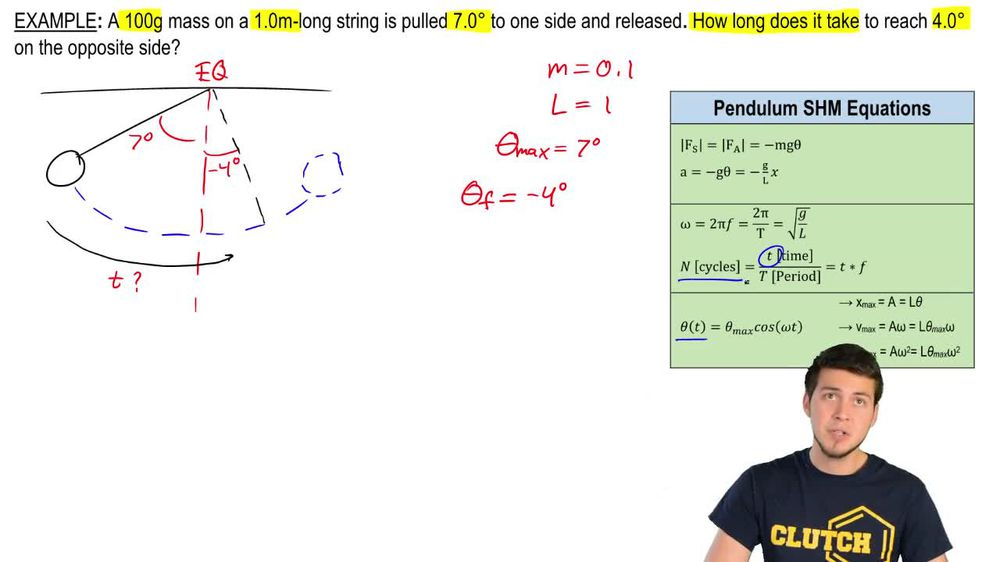Start typing, then use the up and down arrows to select an option from the list.
1. 17. Periodic Motion2. Simple Harmonic Motion of Pendulums# Example

by Patrick Ford
107 views
2
3
2
Hey, astronauts, let's do an example. So this is actually really common type of problem with pendulums your landing on an unfamiliar planet. You're constructing a simple pendulum. So let's go and check it out. We've got this pendulum that's a length of 3 m and a mass of 4 kg. So I've got l equals three and Mass is equal to four. Now, we're gonna release this pendulum. We're gonna pull it back to 10 degrees from the vertical right there, and they were gonna let it go. So it's just gonna oscillate back and forth. So that's our initial push or pull. That's Data Max, and that's equal to 10 degrees. Uh, just remember that because this is in degrees, anytime we're gonna be working with data we're gonna have in radiance. So just make just be aware that you might have to do some conversions, okay? And the last thing we're told is that it's one full cycle at two seconds. What does that mean? It takes two seconds for this thing to go back and then all the way back to the other side work started from So that is the full period, right? That is t equals two seconds. And now what we're supposed to do is calculate the acceleration due to gravity at the surface of this planet. What does that mean? Well, I just want you to be aware of this E Just remember that in Earth G equals 9.8. You probably know that by now. But that any other planet, we cannot take that for granted. So and any other planets G does not equal 98 Right? So just be aware of that. Sometimes you just look at Gene, you're like, Oh, that's 9.8, but it's not in these situations. Okay, So which equation or we're gonna use because we actually have a lot of them involved. So I've got one here. I've got one here, and I've got one here. Well, let's take a look at all my variables. I know the length of the pendulum. I'm told the mass. I'm told the period, and I'm told the Theta Max. Okay, we've got mass here, but I have the theta. Okay, But its data Max. Okay, so it might be able to use it here, but I don't know how the restoring force, so I wouldn't be able to solve Fergie. And in this case, I also don't have the acceleration. So it means I can't use the force of the acceleration because I'm not given that information, Theo. Nly other equation that I could use is this big Omega equation involving squared of D over L. So if I'm trying to figure out what G of the planet is, I'm gonna go ahead and use that big omega equation, so I'm gonna write it out. So you've got square root of G over l. Okay, and we're looking for what the gravity on this particular planet is. So again, what do we have? Well, I have the length of the pendulum, and I also have the period, so I can actually just go ahead and use these last two equations because I only have one unknown. So let me go ahead and write that out. So I got to pie divided by the period. The periods equal to two seconds equals the square root of the gravity on that planet, divided by the length of the pendulum, which is what we're given that it's three. So let's go and do some simplification. We've got the twos. Cancel You got pi equals square root of G over three. So now, in order to get rid of the square root, it's gotta square. Both sides got pi squared, equals G planted over three. And so then I just gotta move the three over so the G planet is equal to three pi squared. So that's equal to 29.6 m per second squared, which is much higher than our 9.8. Okay, so that's it for this one. Let me know if you guys have any issues and let's keep going.